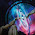## Thursday, November 12, 2009

### Iterator.Sliding

This topic requires Scala 2.8 or higher.

Iterator and Iterable have most of the most useful methods when dealing with collections. Fold, Map, Filter are probably the most common. But other very useful methods include grouped/groupBy, sliding, find, forall, foreach, and many more. I want to cover Iterator's sliding method.

The `def sliding[B >: A](size : Int, step : Int) : GroupedIterator[B]` method provides a sliding window on the iterator. The first parameter is the size of the window and the second is the number of elements to skip each time the window is moved. If step == size then this method is the same as grouped. Step defaults to one

Examples:
`// notice resulting lists are all 3 long and increases by 1scala> (1 to 5).iterator.sliding(3).toListres18: List[Sequence[Int]] = List(List(1, 2, 3), List(2, 3, 4), List(3, 4, 5))// step is 3 so now there are only 2 groupsscala> (1 to 5).iterator.sliding(4, 3).toListres19: List[Sequence[Int]] = List(List(1, 2, 3, 4), List(4, 5))// withPartial(false) makes the partial group be ignoredscala> (1 to 5).iterator.sliding(4, 3).withPartial(false).toListres21: List[Sequence[Int]] = List(List(1, 2, 3, 4))scala> val it2 = Iterator.iterate(20)(_ + 5)it2: Iterator[Int] = non-empty iterator// withPadding fills in the partial group// notice with padding is by-name (it is executed each time it is called)scala> (1 to 5).iterator.sliding(4, 3).withPadding(it2.next).toListres23: List[Sequence[Int]] = List(List(1, 2, 3, 4), List(4, 5, 20, 25))`

1.I know this is a really old post, but I have to leave a comment just to say thanks :).

2.Nice description of sliding.

The examples are from the Scala docs: http://www.scala-lang.org/api/2.11.1/index.html#scala.collection.Iterator\$GroupedIterator

3.Brilliant, thanks!

4.What's the computational complexity of this sliding iterator?

5.What's the computational complexity of this sliding iterator?